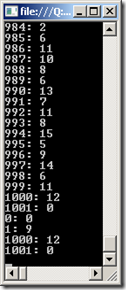## Thursday, July 21, 2011

### Random number generator from binary input

This question is likely if you are dealing with quantum randomizers, etc. It’s irrelevant if there is an appropriate function in the language.

You are given a function that generates 0 and 1 with equal probability. Write a function that uses the above function to generate any n(1<=n<=1000) so that probability of producing any number between 1 to 1000 is equal and is 1/1000.

The solution is simple and fast using bit shifting (which gives performance, *2 could be used but that will raise flags with many interviewers)

Code Snippet
1. static Random _Ran = new Random();
2. public static int ZeroOne()
3. {
4.   return _Ran.Next(2);
5. }
6. /// <summary>
7. /// We bitshift for optimal performance
8. /// </summary>
9. /// <returns></returns>
10. public static int Random1to1000()
11. {
12.   var num = 0;
13.   var outofrange = true;
14.   while (outofrange)
15.   {
16.     num = ZeroOne() << 1;
17.     for (int i = 0; i < 8; i++)
18.     {
19.       num = (num | ZeroOne()) << 1;
20.     }
21.     num = num | ZeroOne();
22.     outofrange = num > 1000 || num < 1;
23.   }
24.   return num;
25. }

Often the ZeroOne would be put internal to the Random1to1000().  If this is done, then to ask for it to be moved to an external function ZeroOne() will usually reveal whether there is understanding of Random issues (i.e. initializing Random inside of ZeroOne will not only result in poorer performance, but will also loose randomness!).

The testing is simple:

Test Code
1. var cnt = new int;
2. for (var i = 1; i < 10000; i++)
3. {
4.   var num = Questions.Random1to1000();
5.   cnt[num]++;
6. }
7. for (var i = 0; i < cnt.Length; i++)
8. {
9.   Console.WriteLine(string.Format("{0}: {1}", i, cnt[i]));
10. }
11. Console.WriteLine(string.Format("{0}: {1}", 0, cnt));
12. Console.WriteLine(string.Format("{0}: {1}", 1, cnt));
13. Console.WriteLine(string.Format("{0}: {1}", 1000, cnt));
14. Console.WriteLine(string.Format("{0}: {1}", 1001, cnt));

The results allows spot testing:Often people will assume that zero(0) will not occur from the code (to see a lot of code that allows a zero to be returned, see this).